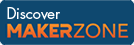Cody

# Problem 1072. Television Screen Dimensions

Given a width to height ratio of a TV screen given as w and h as well as the diagonal length of the television l, return the actual width and height of the screen to one decimal point of precision.

Example:

```    w = 16;
h = 9;
l = 42;
[W,H] = teledims(w,h,l);```

outputs

```    W = 36.6
H = 20.6```

### Solution Stats

37.93% Correct | 62.07% Incorrect
Last solution submitted on Mar 26, 2019

#### TagsMATLAB and Simulink resources for Arduino, LEGO, and Raspberry Pi